Function Repository Resource:

# RoundedCuboid

Represent a cuboid with rounded edges

Contributed by: Jaroslav Kysela
 ResourceFunction["RoundedCuboid"][pmin] represents a unit cube with rounded edges and with its lower corner at pmin. ResourceFunction["RoundedCuboid"][pmin,pmax] represents an axis-aligned cuboid with rounded edges and with lower corner pmin and upper corner pmax.

## Details and Options

ResourceFunction["RoundedCuboid"] can be used in Graphics3D.
Graphics rendering is affected by directives such as FaceForm, Opacity, and color.
ResourceFunction["RoundedCuboid"][] is equivalent to ResourceFunction["RoundedCuboid"][{0,0,0}].
The following options can be given:
The RoundingRadius setting can have the following forms:
 r use r for radii in all three directions {rx,ry,rz} separate setting for each radius

## Examples

### Basic Examples (4)

A unit rounded cuboid:

 In:=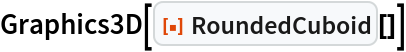Out=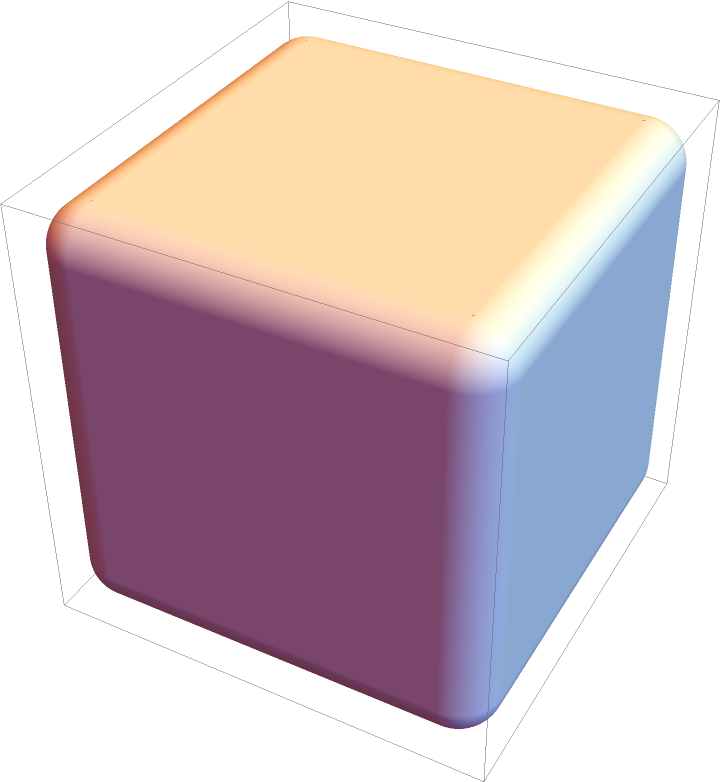Rounded cuboids with different sizes:

 In:=Out=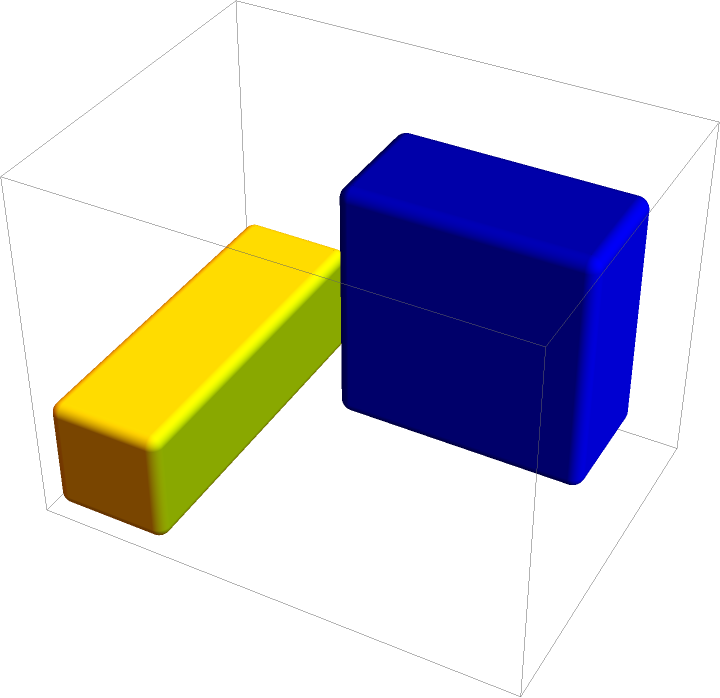Differently styled rounded cuboids:

 In:=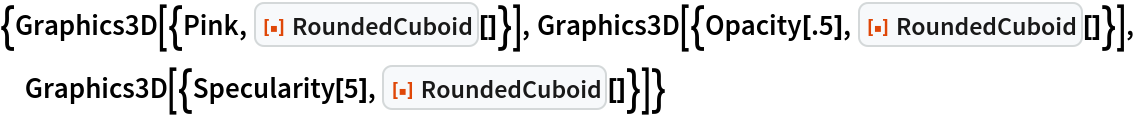Out=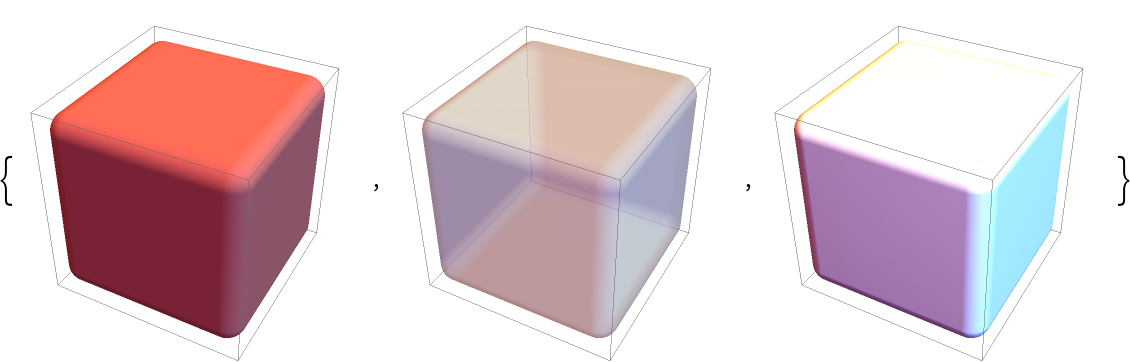Rotated rounded cuboid:

 In:=Out=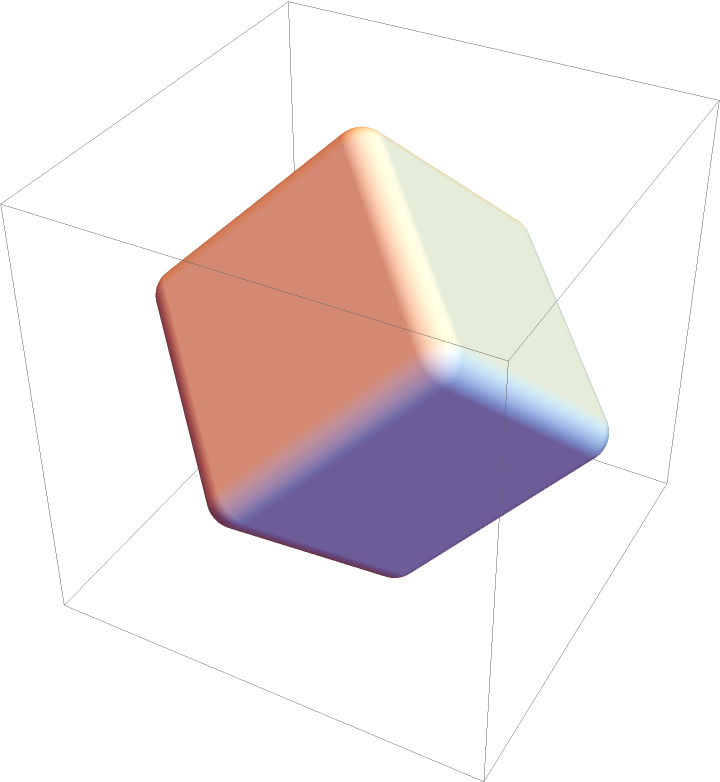### Scope (8)

#### Specification (3)

A rounded unit cube:

 In:=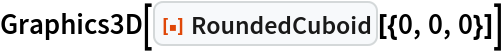Out=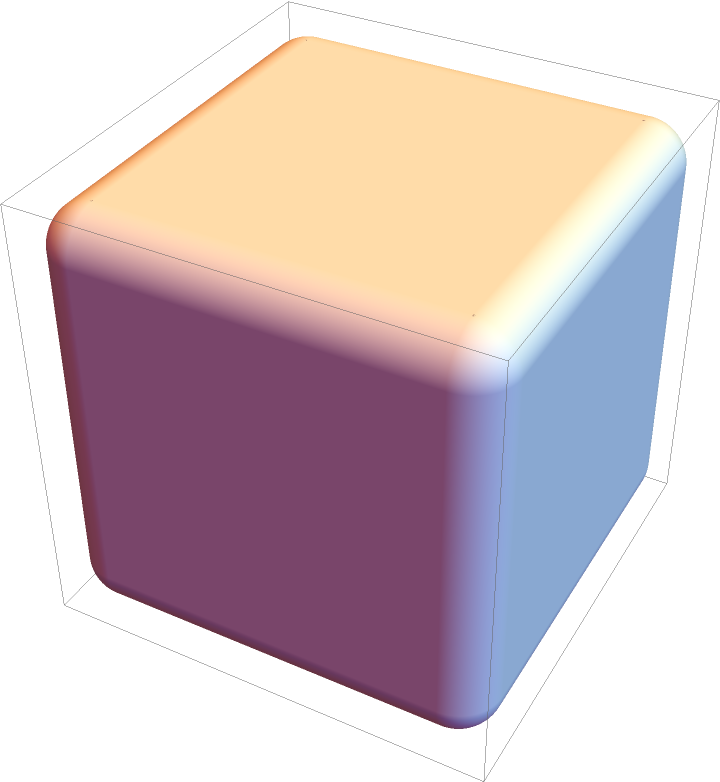A rounded cuboid parallel to each axis:

 In:=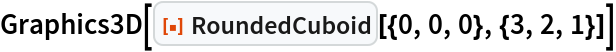Out=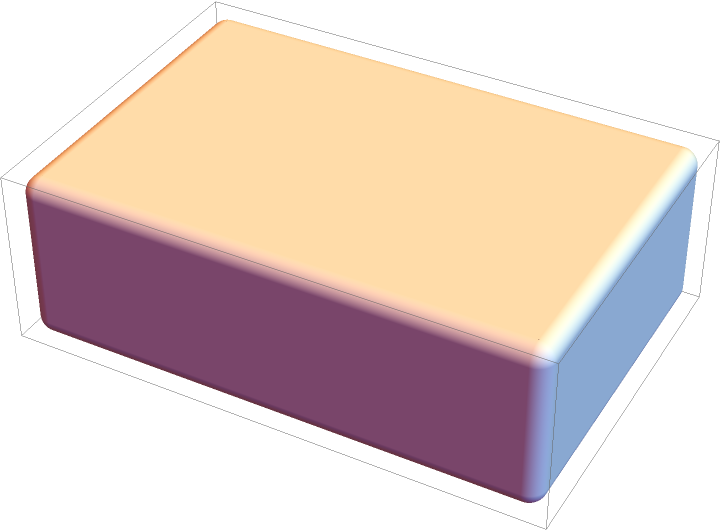Short form for a rounded unit cube cornered at the origin:

 In:=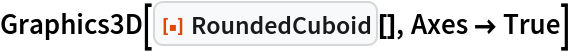Out=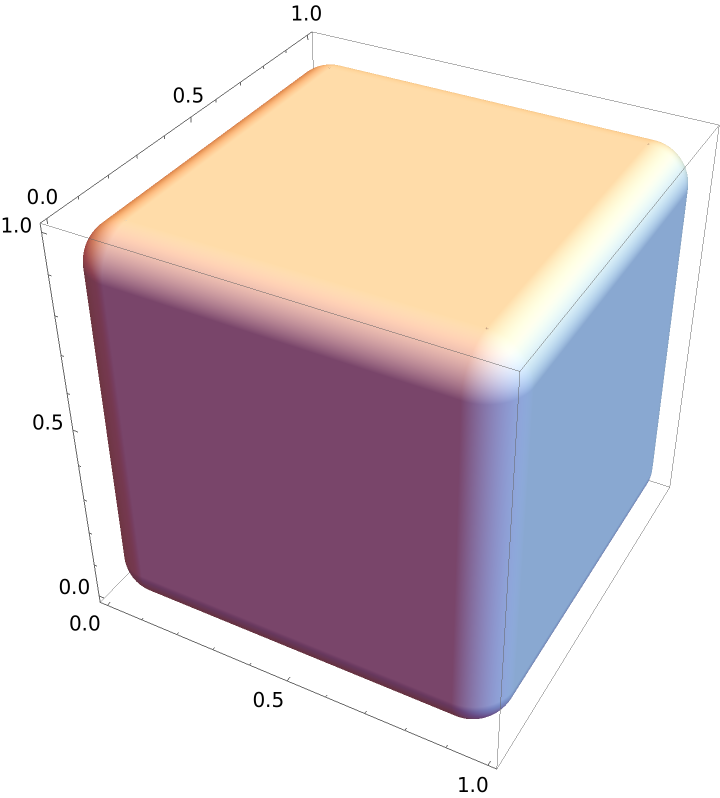#### Styling (5)

Color directives specify the face colors of cuboids:

 In:=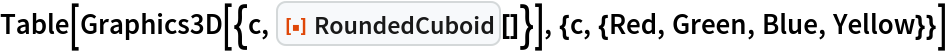Out=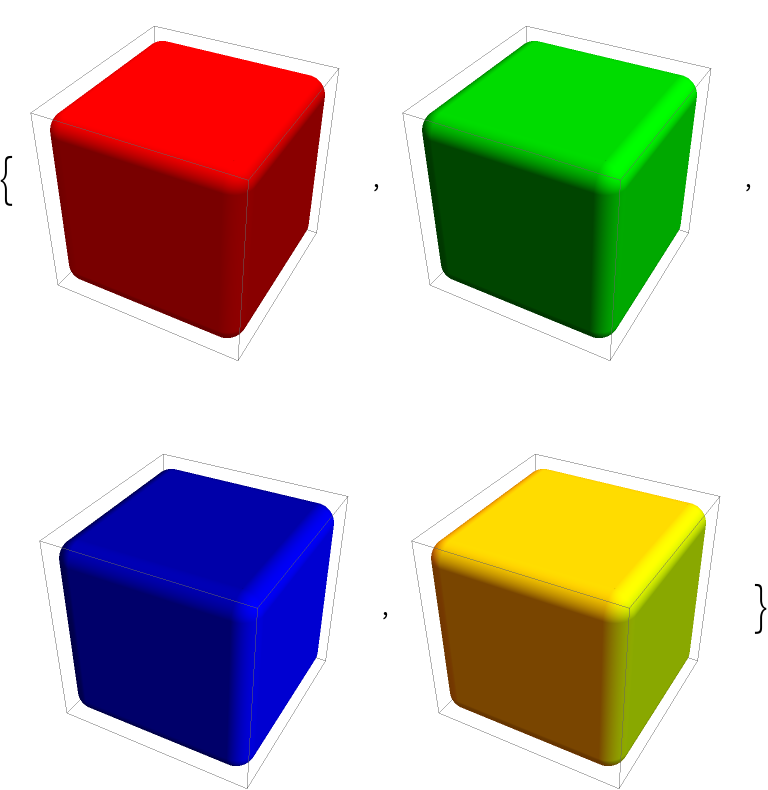FaceForm can be used to specify the styles of the faces:

 In:=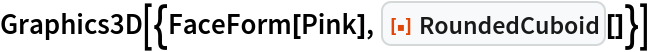Out=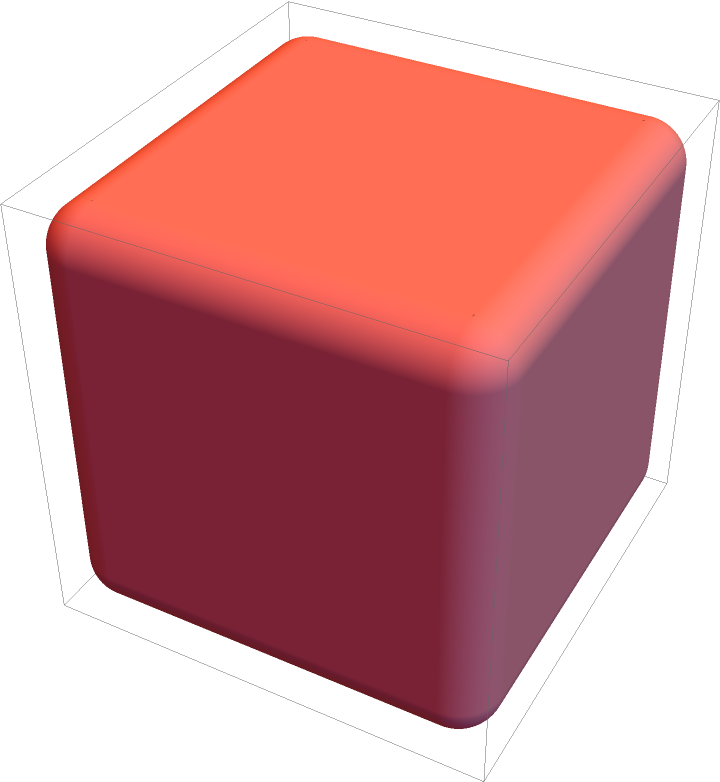Different properties can be specified for the front and back of faces using FaceForm:

 In:=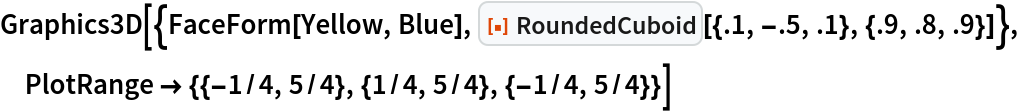Out=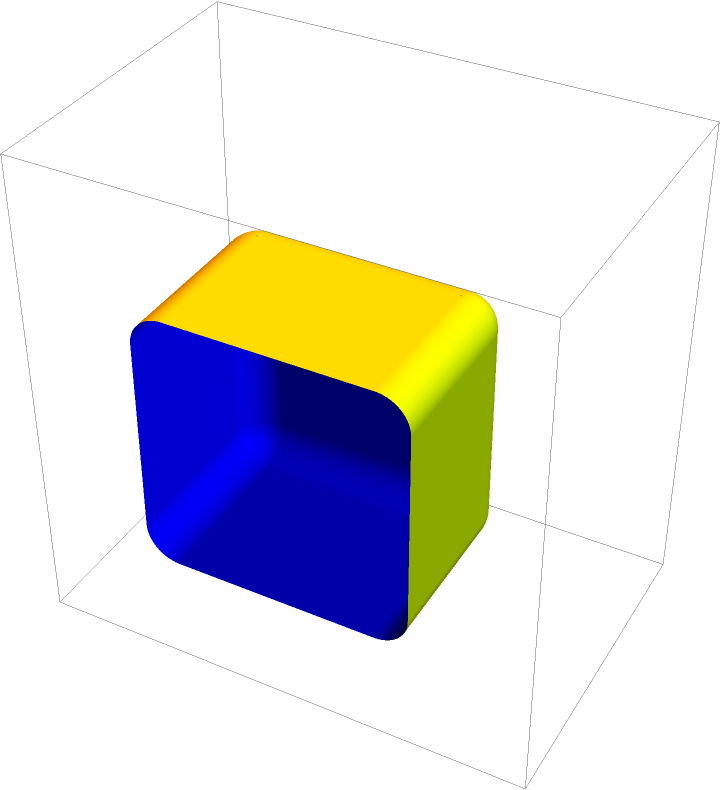Opacity specifies the face opacity:

 In:=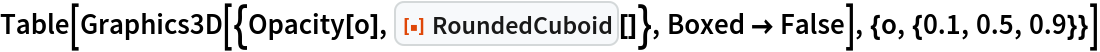Out=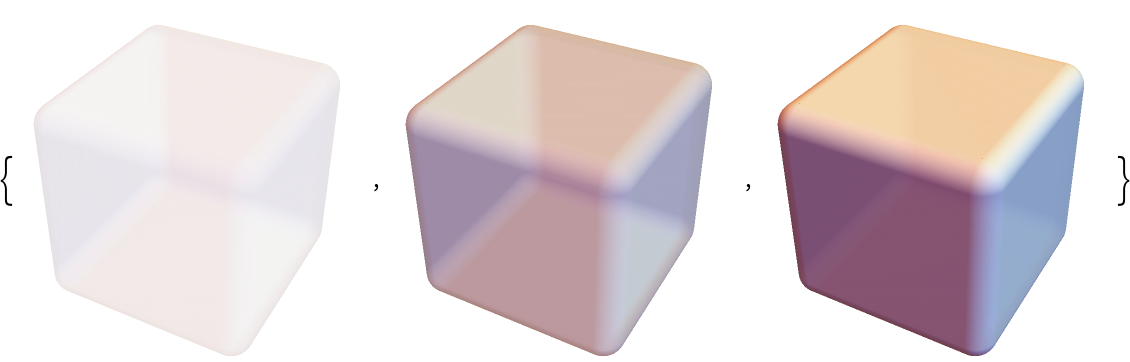Decompose the cuboid into its components and apply different styling to each:

 In:=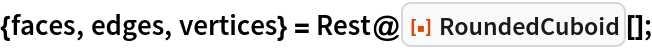In:=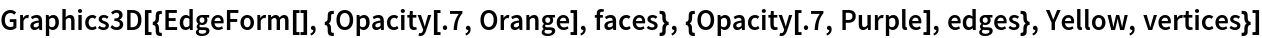Out=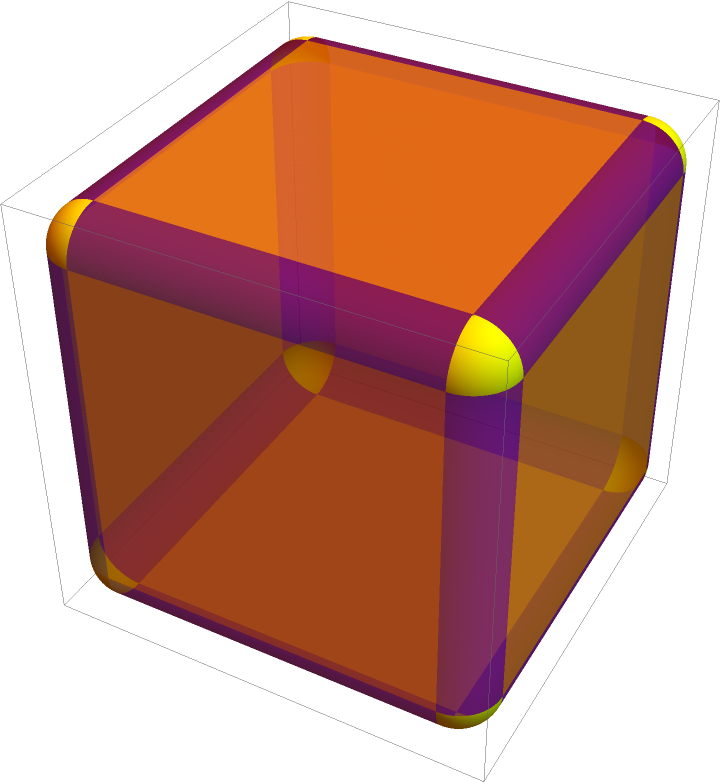### Options (3)

Adjust how rounded the edges are:

 In:=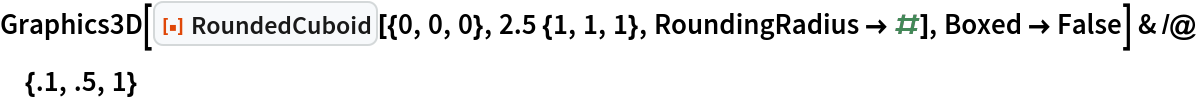Out=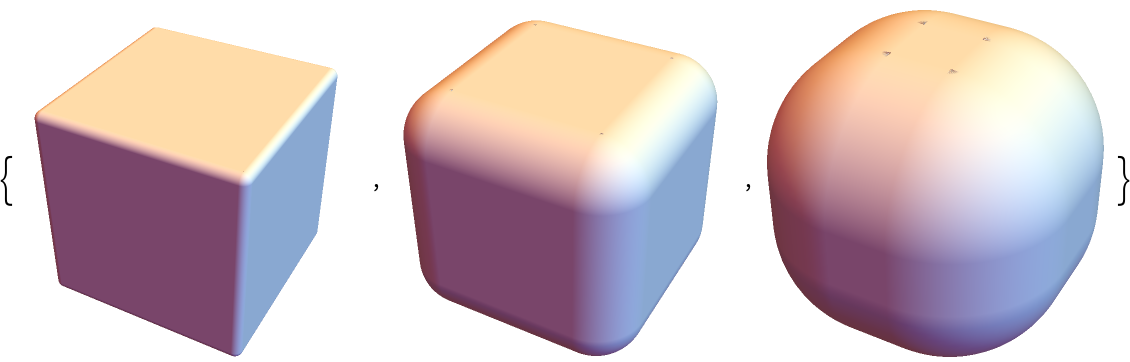Adjust the edges in each direction independently:

 In:=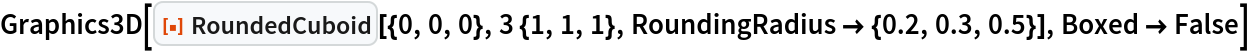Out=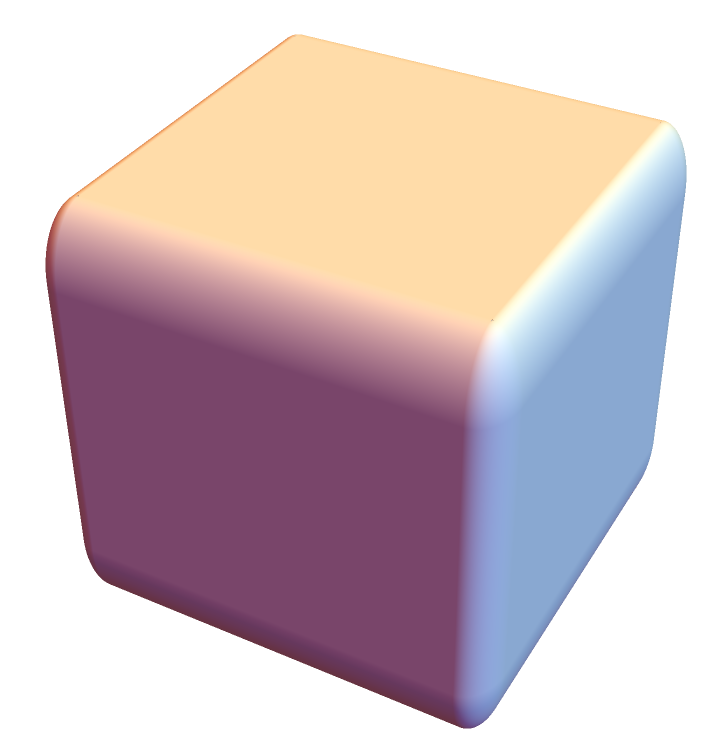Leave some edges sharp:

 In:=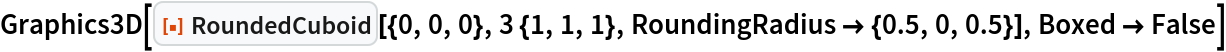Out=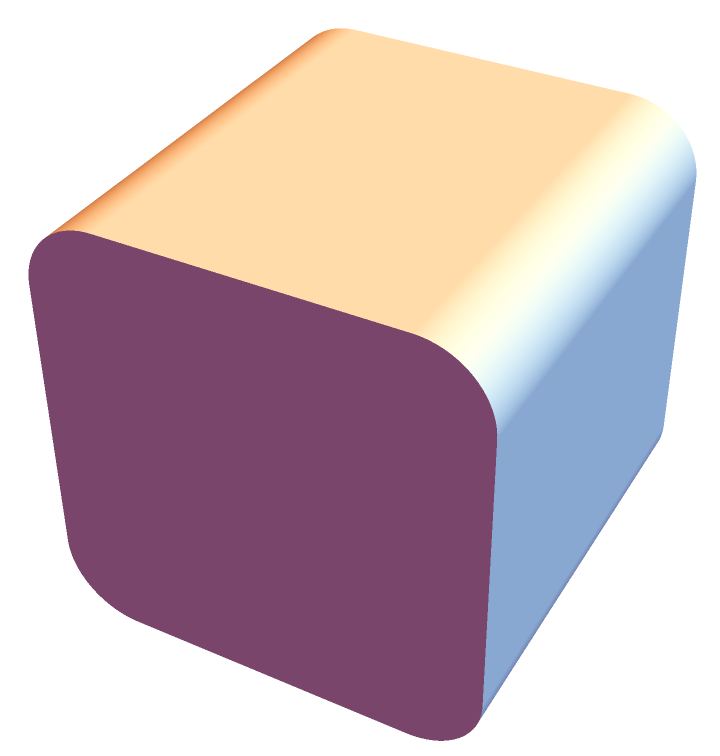### Applications (1)

A simple 3D bar chart:

 In:=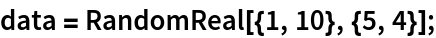In:=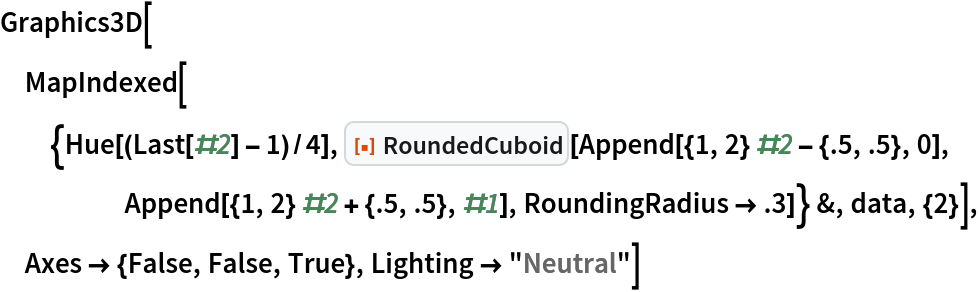Out=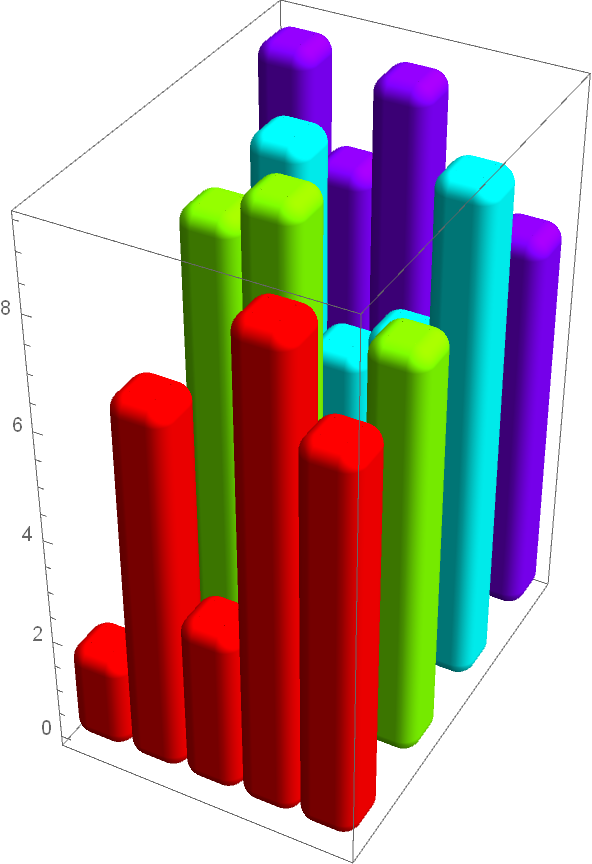### Properties and Relations (7)

Use Rotate to get all possible rounded cuboids in Graphics3D:

 In:=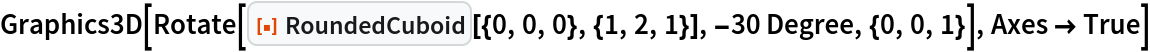Out=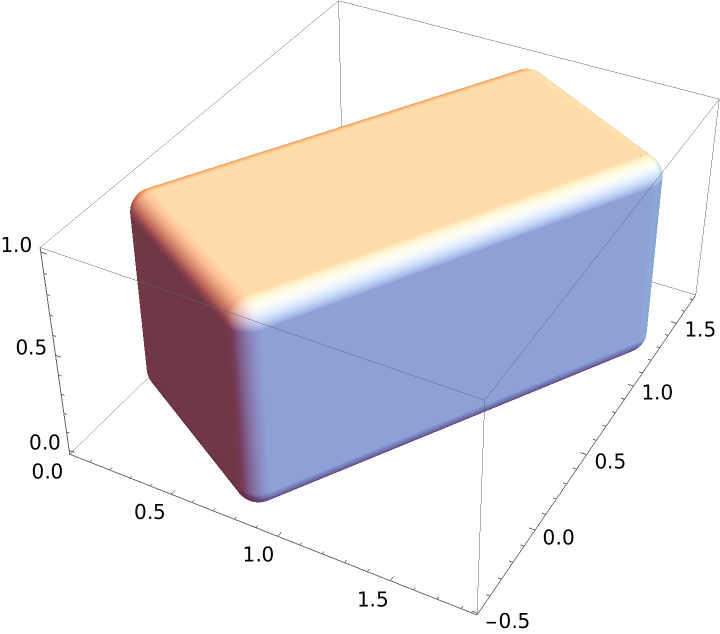Rectangle with a non-zero value for RoundingRadius is a 2D version of RoundedCuboid:

 In:=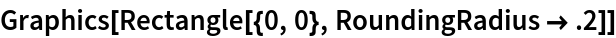Out=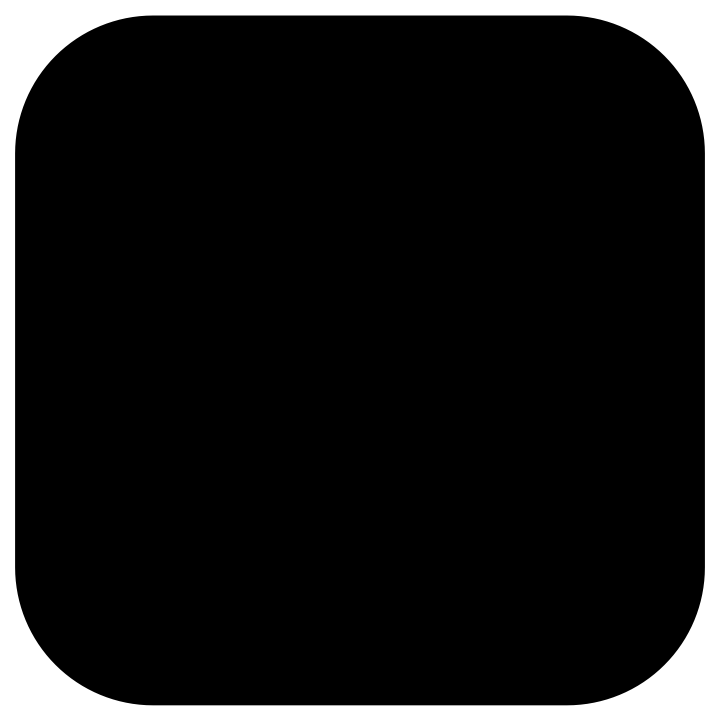Cuboid is a special case of RoundedCuboid:

 In:=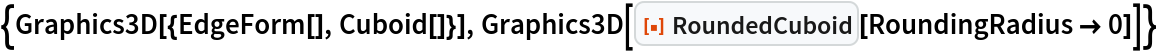Out=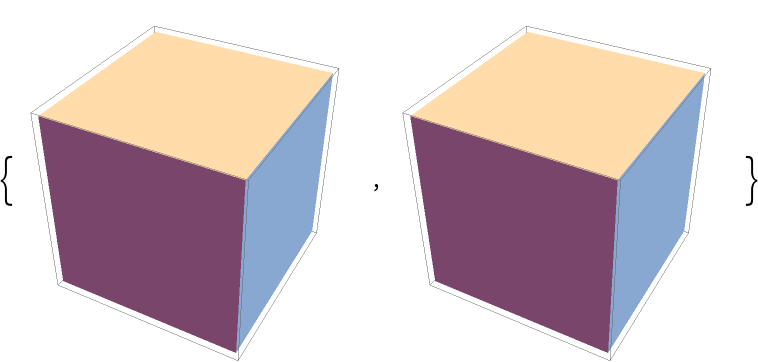Cylinder can be viewed as a special case of RoundedCuboid:

 In:=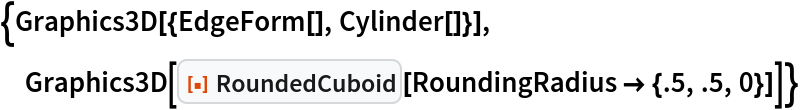Out=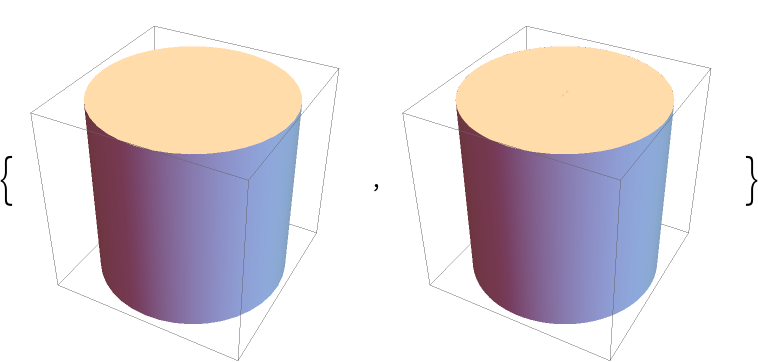Sphere can be viewed as a special case of RoundedCuboid:

 In:=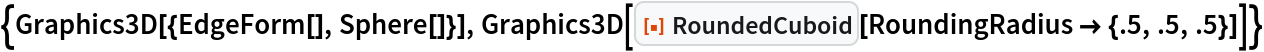Out=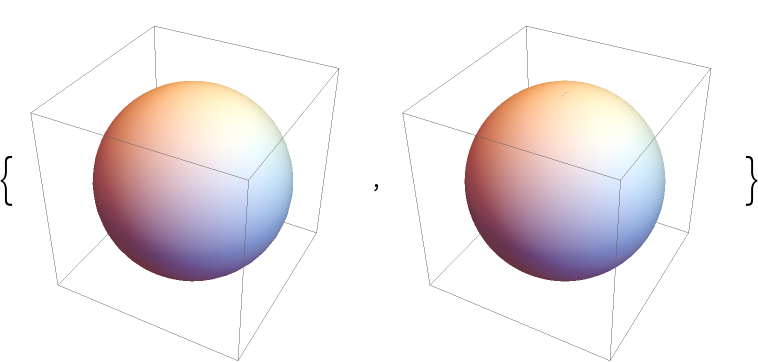BarChart3D or RectangleChart3D can be used to create rounded cuboids:

 In:=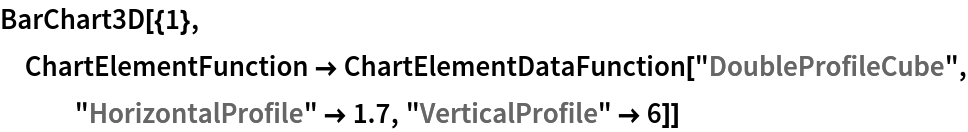Out=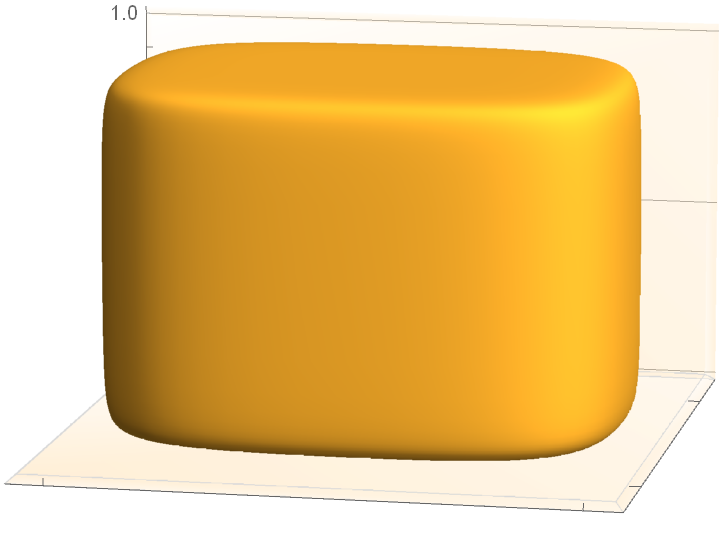Get the graphical primitive for BarChart3D:

 In:=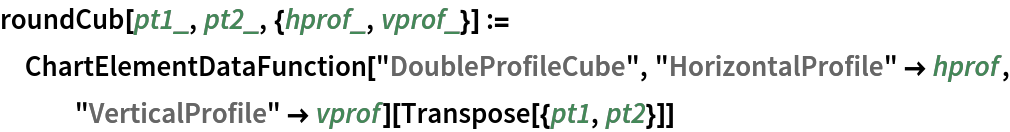In:=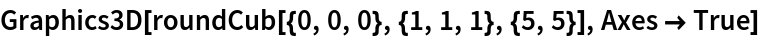Out=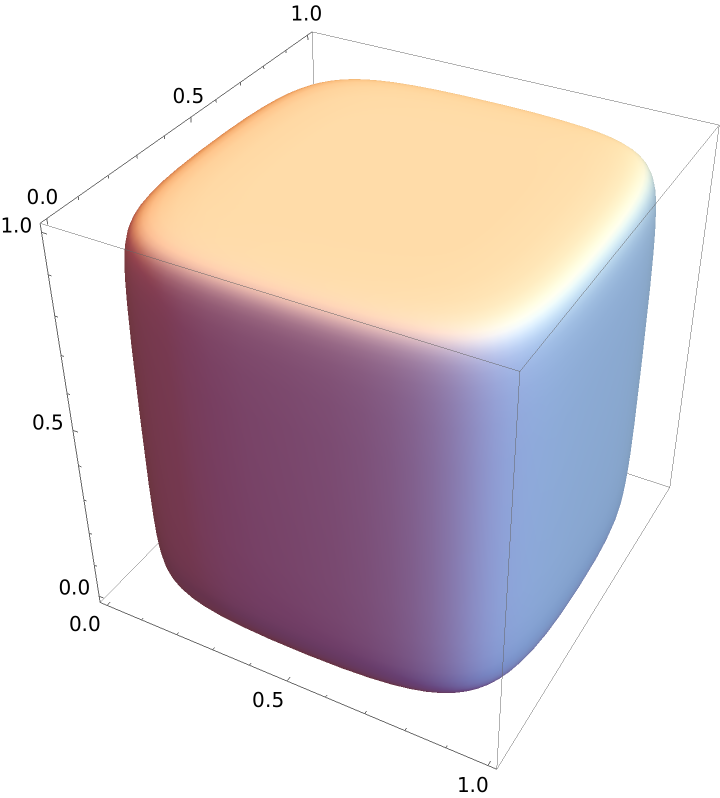Compare with RoundedCuboid:

 In:=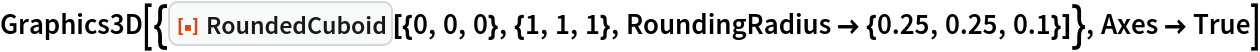Out=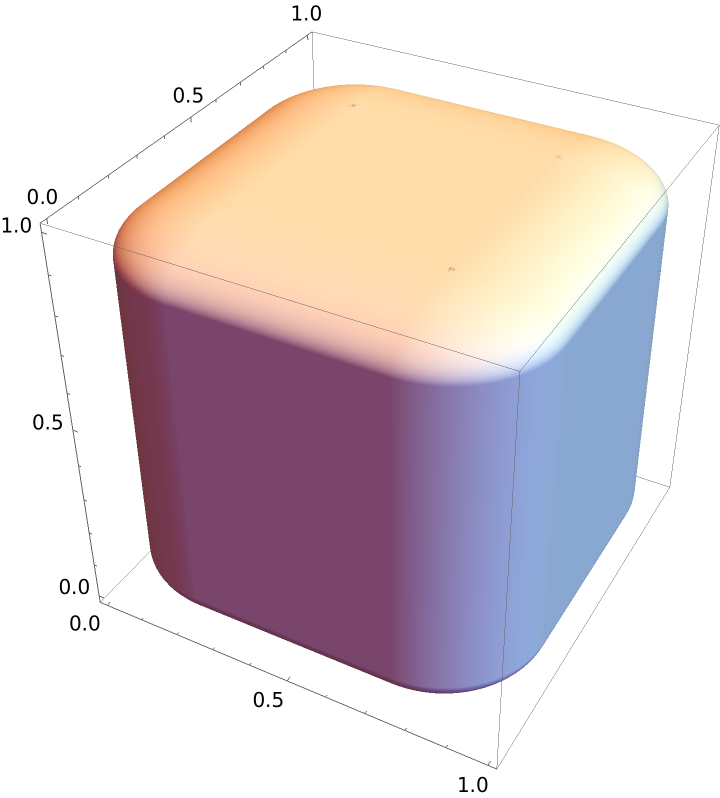The two cuboids have a very different internal structure:

 In:=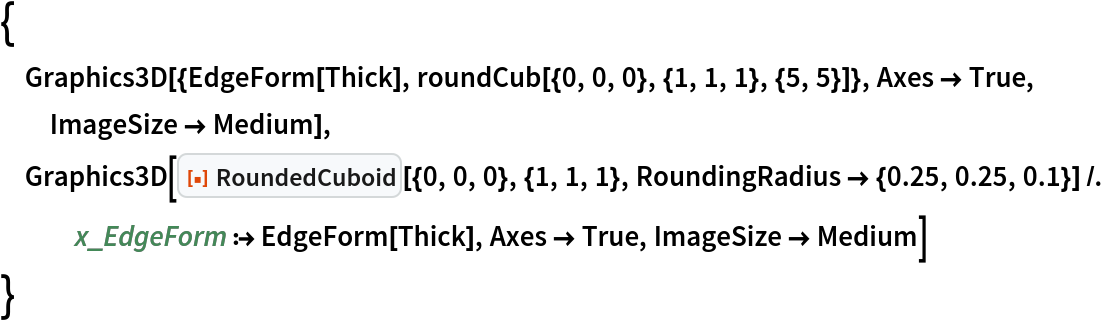Out=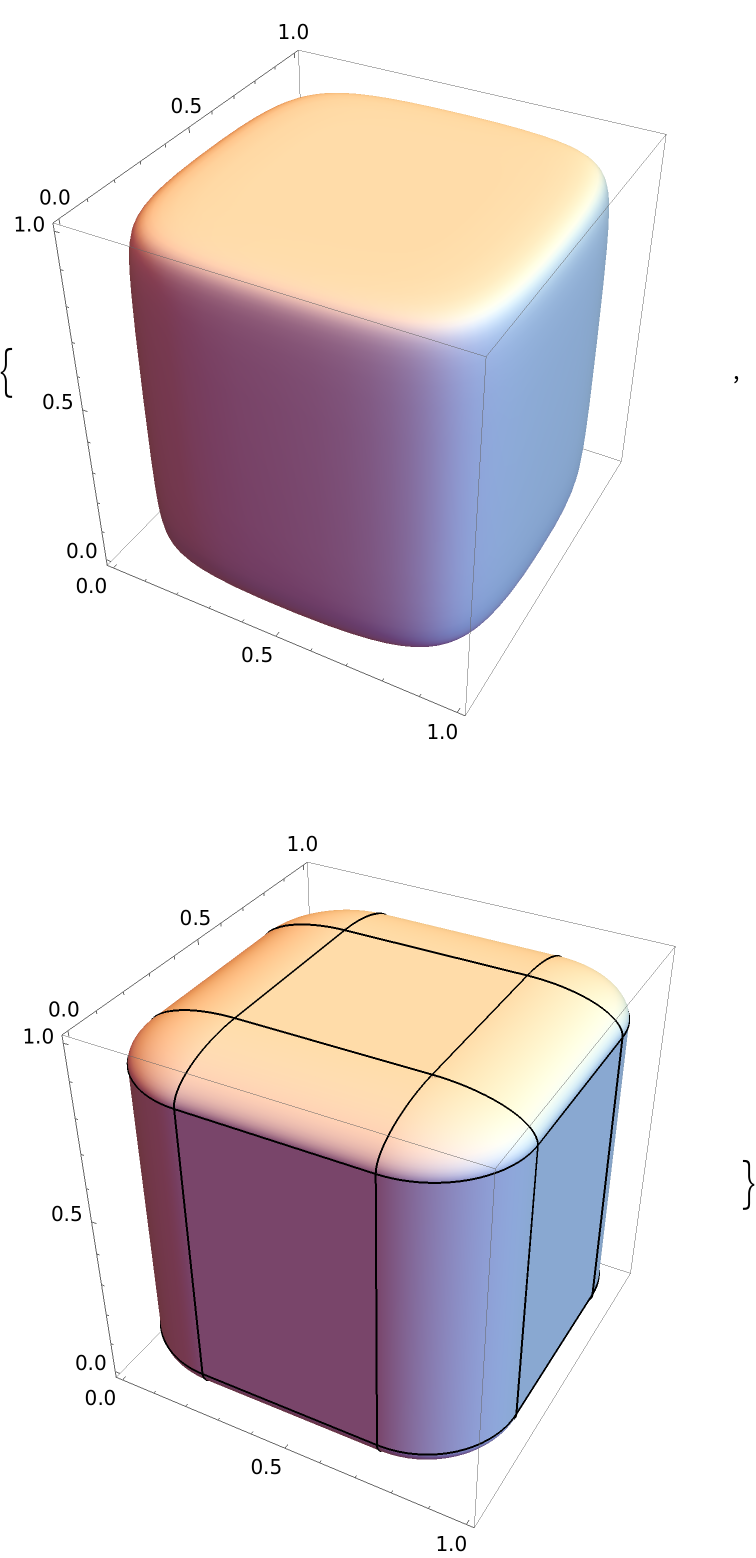### Possible Issues (2)

When the width of the cuboid is zero in at least one dimension, no cuboid is created:

 In:=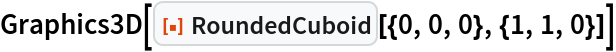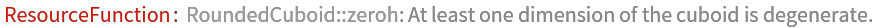Out=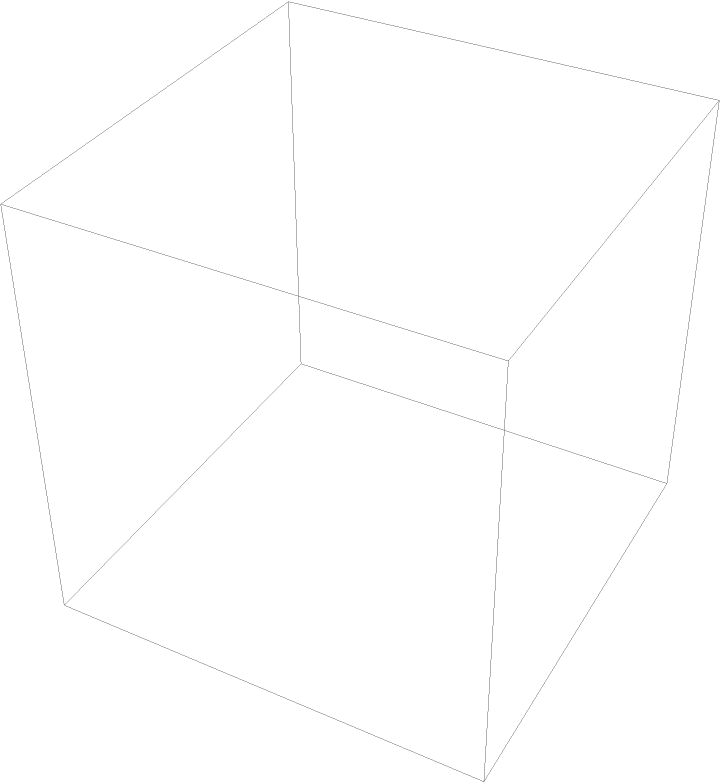When any length of the cuboid is less than twice the corresponding setting for RoundingRadius, a warning is issued and artifacts appear:

 In:=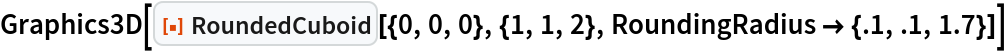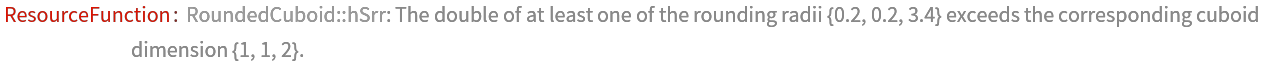Out=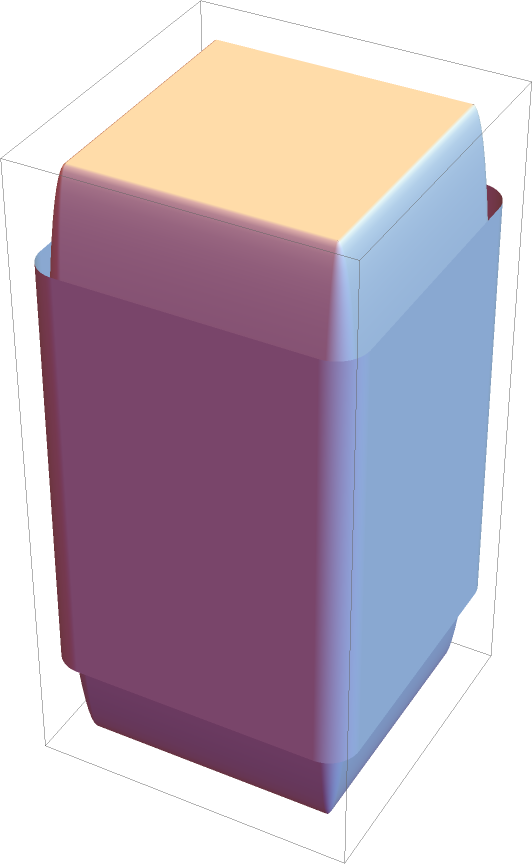### Neat Examples (2)

Random rounded cuboid collections:

 In:=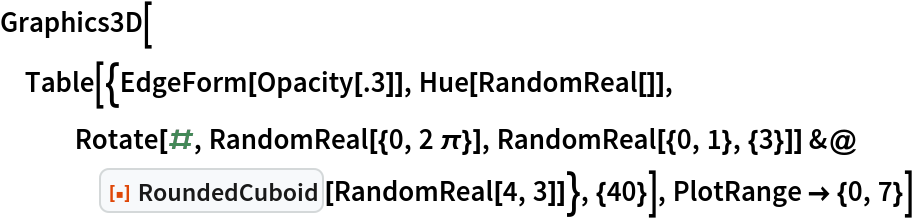Out=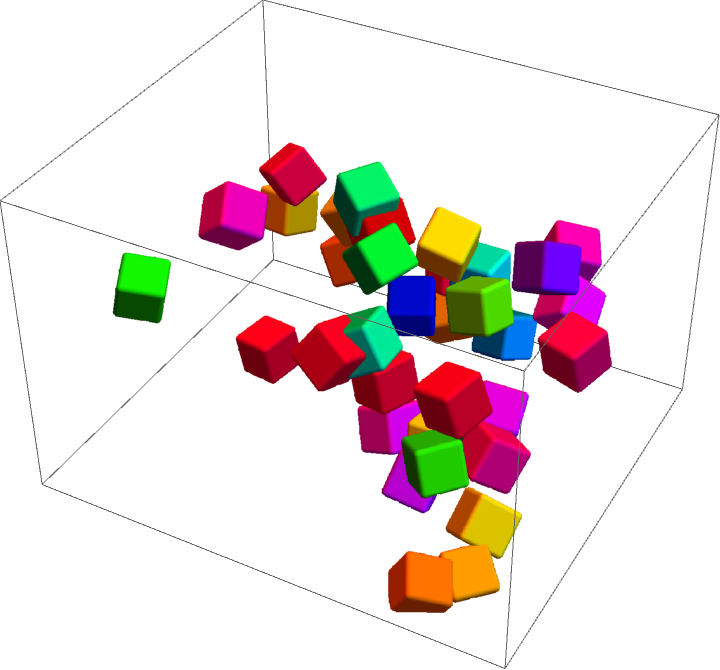In:=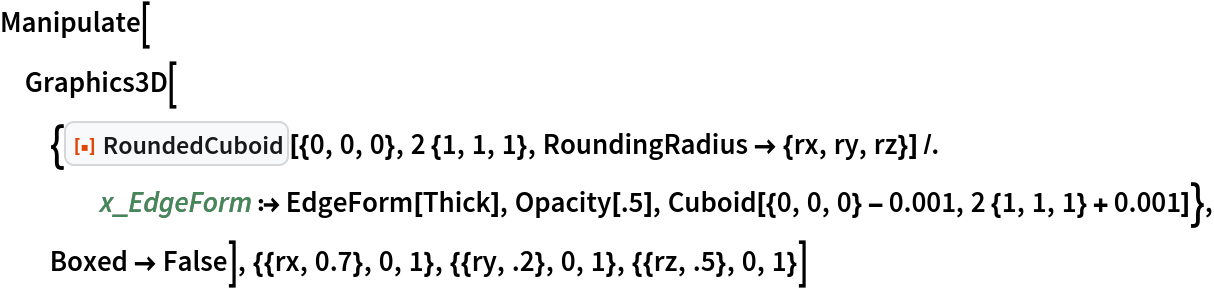Out=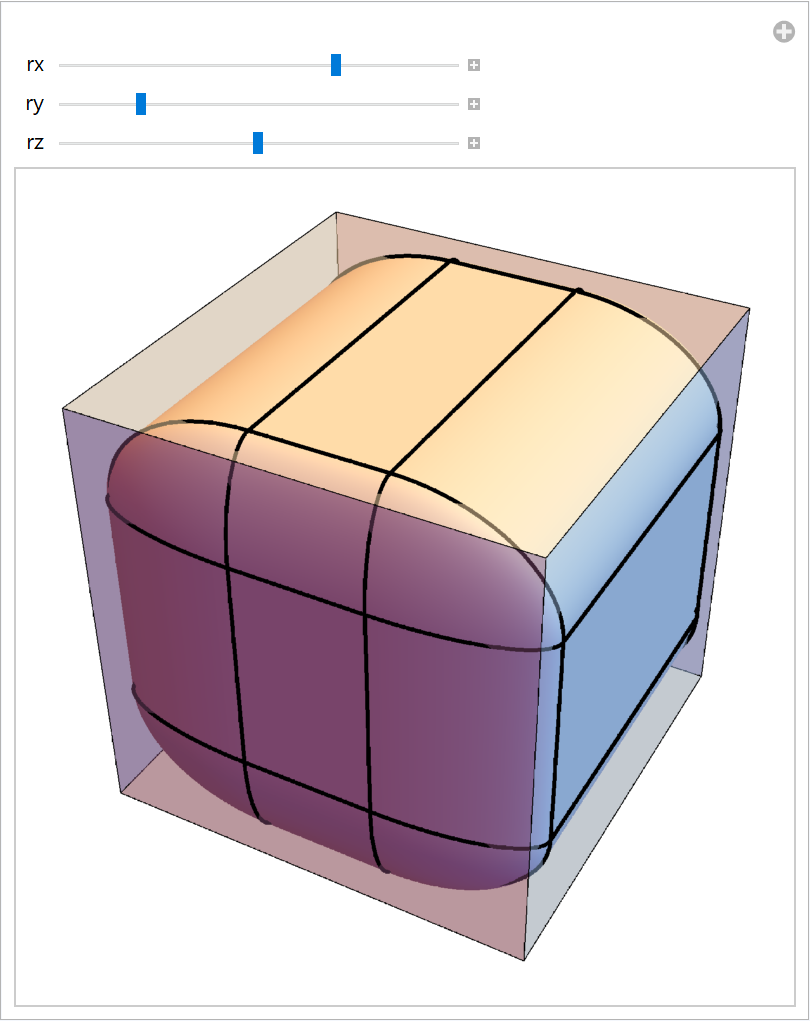Jaroslav Kysela

## Version History

• 1.0.0 – 03 January 2022